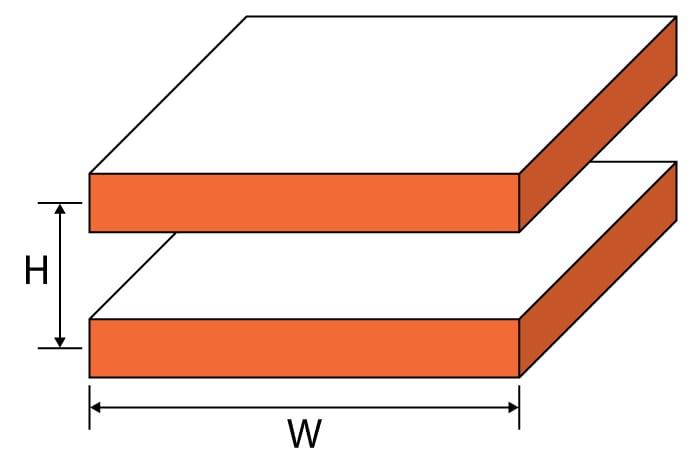# Broadside Coupled Trace Inductance Calculator

## This calculator helps you compute the inductance of a broadside coupled trace.

H

H/m

### Overview

This calculator will help you calculate the inductance of a broadside coupled trace given its dimensions. It is common to find the broadside coupled trace in printed circuit boards where differential pair signals are routed. Traces such as these are found on adjacent planes with the return being identical to the trace both in length and in width. In the industrial setting, this routing techique is done to ensure strong coupling although the actual return for the signal is commonly on the power plane.### Equation

$$L_{bs}=\frac{\mu_{0} \mu_{r} H}{W}$$

Note:

$$W >> H, H > T$$

Where:

$$W$$ – trace width
$$T$$ – trace thickness
$$H$$ – distance between traces
$$L$$ – trace length

### Applications

It is important for an engineer to calculate the inductance between two traces in a printed circuit board as it contributes to the overall circuit's performance.  As the cost in the production of printed circuit boards increases, manufacturers are forced to used higher density routing of high speed signal traces. This can only result in increased crosstalk among the traces. If the inductance of the broadside trace can be determined beforehand, then necessary adjustments can be made.